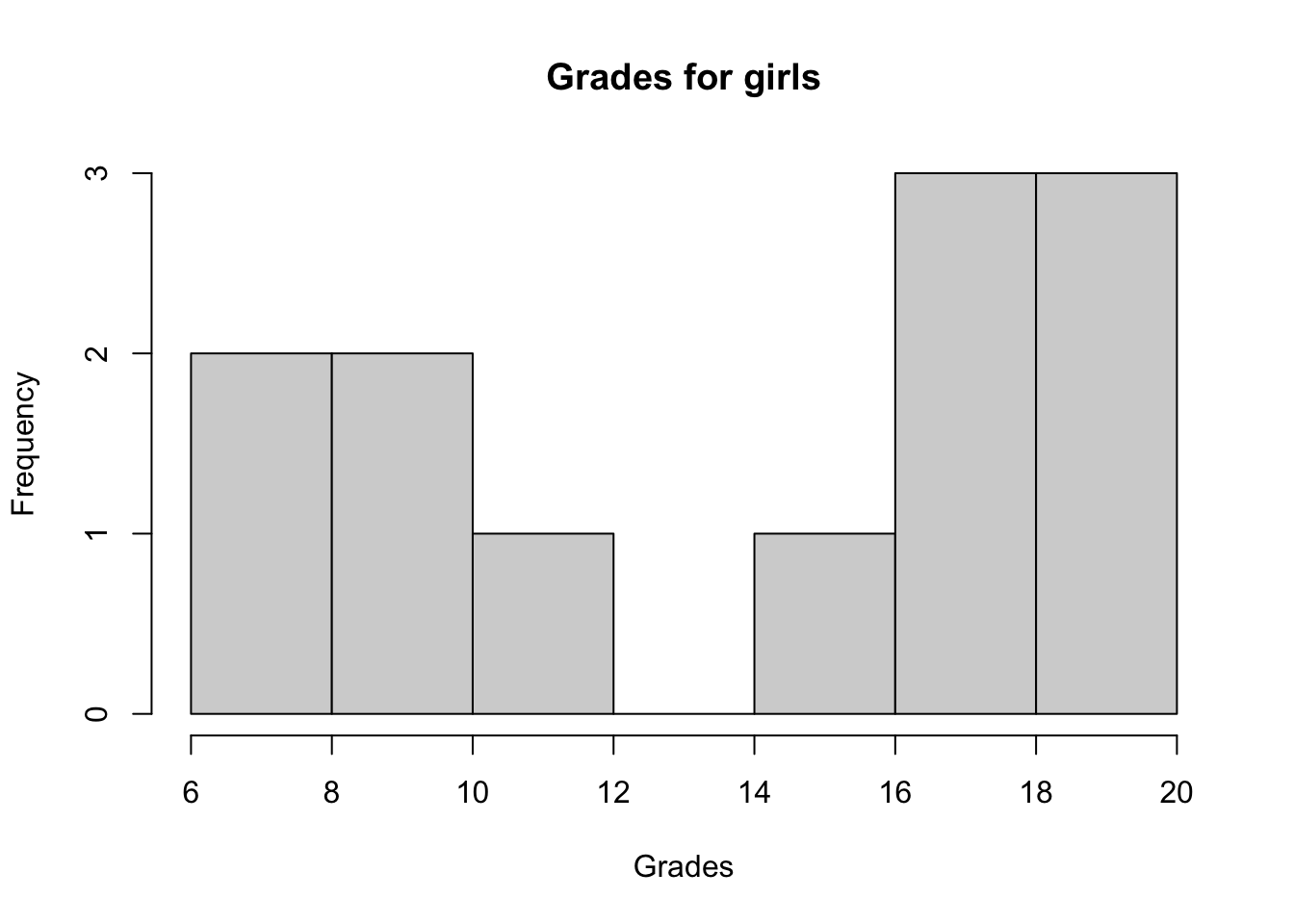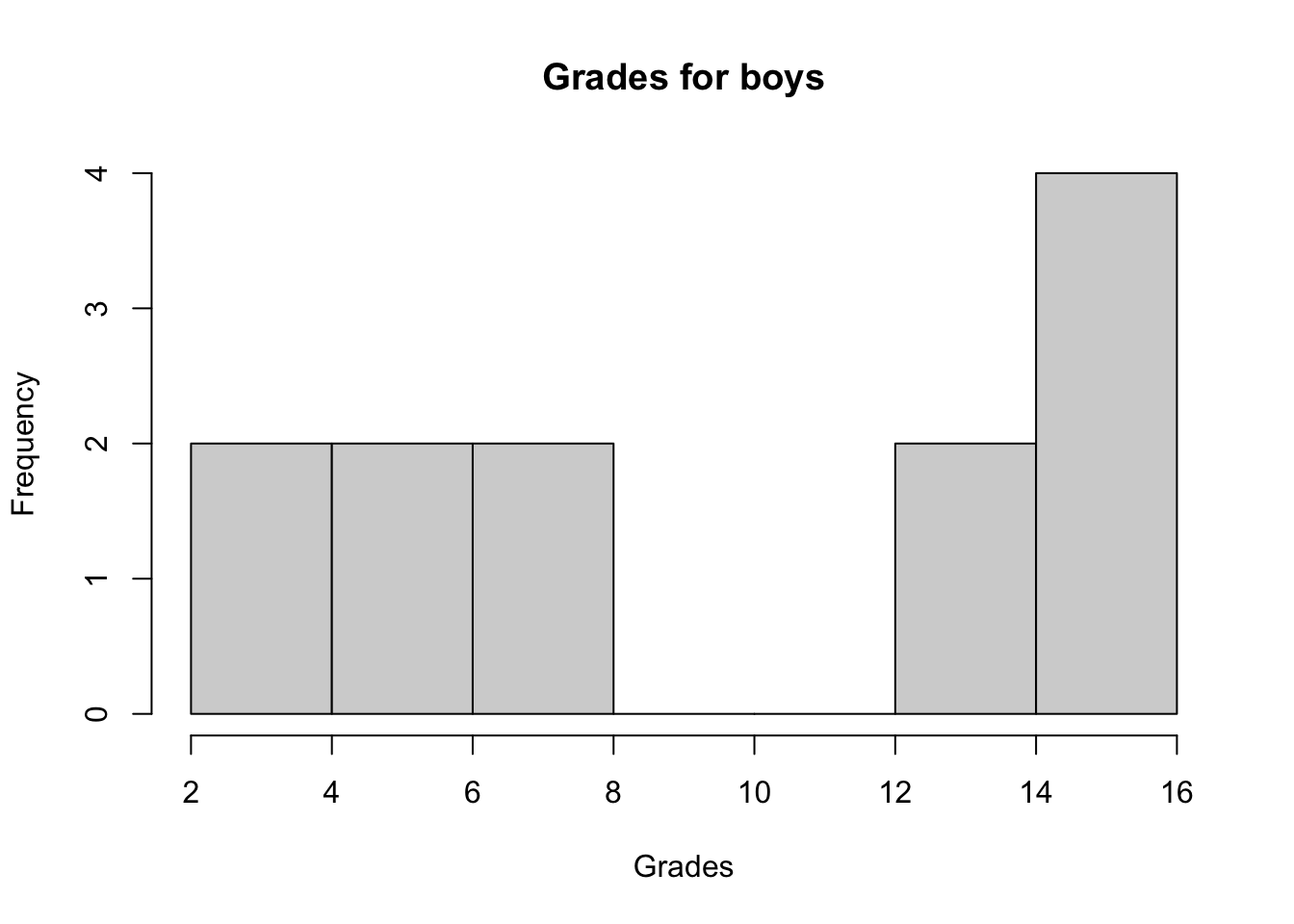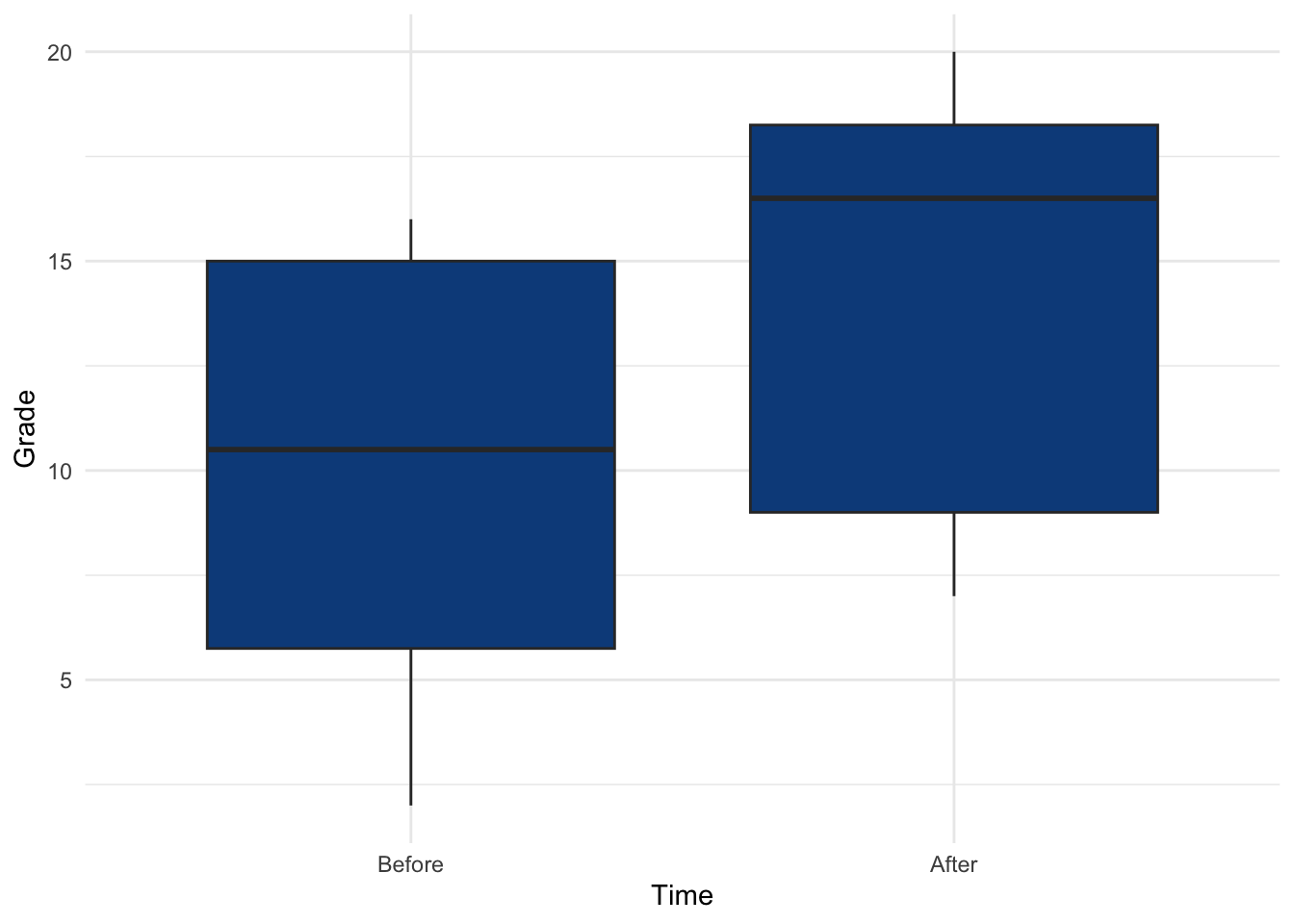# Wilcoxon test in R: how to compare 2 groups under the non-normality assumption# Introduction

In a previous article, we showed how to compare two groups under different scenarios using the Student’s t-test. The Student’s t-test requires that the distributions follow a normal distribution.1 In this article, we show how to compare two groups when the normality assumption is violated, using the Wilcoxon test.

The Wilcoxon test (also referred as the Mann-Withney-Wilcoxon test) is a non-parametric test, meaning that it does not rely on data belonging to any particular parametric family of probability distributions. Non-parametric tests have the same objective as their parametric counterparts. However, they have an advantage over parametric tests: they do not require the assumption of normality of distributions. A Student’s t-test for instance is only applicable if the data are Gaussian or if the sample size is large enough (usually $$n \ge 30$$). A non-parametric should be used in other cases.

One may wonder why we would not always use a non-parametric test so we do not have to bother about testing for normality. The reason is that non-parametric tests are usually less powerful than corresponding parametric tests when the normality assumption holds. Therefore, all else being equal, with a non-parametric test you are less likely to reject the null hypothesis when it is false if the data follows a normal distribution. It is thus preferred to use the parametric version of a statistical test when the assumptions are met.

In the remaining of the article, we present the two scenarios of the Wilcoxon test and how to perform them in R through two examples.

# 2 different scenarios

As for the Student’s t-test, the Wilcoxon test is used to compare two groups and see whether they are significantly different from each other.

The 2 groups to be compared are either:

1. independent, or
2. paired (i.e., dependent)

## Independent samples

For the Wilcoxon test with independent samples, suppose that we want to test whether grades at the statistics exam differ between female and male students.

We have collected grades for 24 students (12 girls and 12 boys):

dat <- data.frame(
Sex = as.factor(c(rep("Girl", 12), rep("Boy", 12))),
19, 18, 9, 17, 8, 7, 16, 19, 20, 9, 11, 18,
16, 5, 15, 2, 14, 15, 4, 7, 15, 6, 7, 14
)
)

dat
##     Sex Grade
## 1  Girl    19
## 2  Girl    18
## 3  Girl     9
## 4  Girl    17
## 5  Girl     8
## 6  Girl     7
## 7  Girl    16
## 8  Girl    19
## 9  Girl    20
## 10 Girl     9
## 11 Girl    11
## 12 Girl    18
## 13  Boy    16
## 14  Boy     5
## 15  Boy    15
## 16  Boy     2
## 17  Boy    14
## 18  Boy    15
## 19  Boy     4
## 20  Boy     7
## 21  Boy    15
## 22  Boy     6
## 23  Boy     7
## 24  Boy    14

Here are the distributions of the grades by sex:

library(ggplot2)

ggplot(dat) +
aes(x = Sex, y = Grade) +
geom_boxplot(fill = "#0c4c8a") +
theme_minimal()We first check whether the 2 samples follow a normal distribution via a histogram and the Shapiro-Wilk test:

hist(subset(dat, Sex == "Girl")$Grade, main = "Grades for girls", xlab = "Grades" )hist(subset(dat, Sex == "Boy")$Grade,
)shapiro.test(subset(dat, Sex == "Girl")$Grade) ## ## Shapiro-Wilk normality test ## ## data: subset(dat, Sex == "Girl")$Grade
## W = 0.84548, p-value = 0.0323
shapiro.test(subset(dat, Sex == "Boy")$Grade) ## ## Shapiro-Wilk normality test ## ## data: subset(dat, Sex == "Boy")$Grade
## W = 0.84313, p-value = 0.03023

The histograms show that both distributions do not seem to follow a normal distribution and the p-values of the Shapiro-Wilk tests confirm it (since we reject the null hypothesis of normality for both distributions at the 5% significance level).

We just showed that normality assumption is violated for both groups so it is now time to see how to perform the Wilcoxon test in R.2 Remember that the null and alternative hypothesis of the Wilcoxon test are as follows:

• $$H_0$$: the 2 groups are similar
• $$H_1$$: the 2 groups are different
test <- wilcox.test(dat$Grade ~ dat$Sex)
test
##
##  Wilcoxon rank sum test with continuity correction
##
## data:  dat$Grade by dat$Sex
## W = 31.5, p-value = 0.02056
## alternative hypothesis: true location shift is not equal to 0

We obtain the test statistic, the p-value and a reminder of the hypothesis tested.3

The p-value is 0.021. Therefore, at the 5% significance level, we reject the null hypothesis and we conclude that grades are significantly different between girls and boys.

Given the boxplot presented above showing the grades by sex, one may see that girls seem to perform better than boys. This can be tested formally by adding the alternative = "less" argument to the wilcox.test() function:4

test <- wilcox.test(dat$Grade ~ dat$Sex,
alternative = "less"
)
test
##
##  Wilcoxon rank sum test with continuity correction
##
## data:  dat$Grade by dat$Sex
## W = 31.5, p-value = 0.01028
## alternative hypothesis: true location shift is less than 0

The p-value is 0.01. Therefore, at the 5% significance level, we reject the null hypothesis and we conclude that boys performed significantly worse than girls (which is equivalent than concluding that girls performed significantly better than boys).

## Paired samples

For this second scenario, consider that we administered a math test in a class of 12 students at the beginning of a semester, and that we administered a similar test at the end of the semester to the exact same students. We have the following data:

dat <- data.frame(
Beginning = c(16, 5, 15, 2, 14, 15, 4, 7, 15, 6, 7, 14),
End = c(19, 18, 9, 17, 8, 7, 16, 19, 20, 9, 11, 18)
)

dat
##    Beginning End
## 1         16  19
## 2          5  18
## 3         15   9
## 4          2  17
## 5         14   8
## 6         15   7
## 7          4  16
## 8          7  19
## 9         15  20
## 10         6   9
## 11         7  11
## 12        14  18

We transform the dataset to have it in a tidy format:

dat2 <- data.frame(
Time = c(rep("Before", 12), rep("After", 12)),
Grade = c(dat$Beginning, dat$End)
)
dat2
##      Time Grade
## 1  Before    16
## 2  Before     5
## 3  Before    15
## 4  Before     2
## 5  Before    14
## 6  Before    15
## 7  Before     4
## 8  Before     7
## 9  Before    15
## 10 Before     6
## 11 Before     7
## 12 Before    14
## 13  After    19
## 14  After    18
## 15  After     9
## 16  After    17
## 17  After     8
## 18  After     7
## 19  After    16
## 20  After    19
## 21  After    20
## 22  After     9
## 23  After    11
## 24  After    18

The distribution of the grades at the beginning and after the semester:

# Reordering dat2$Time dat2$Time <- factor(dat2$Time, levels = c("Before", "After") ) ggplot(dat2) + aes(x = Time, y = Grade) + geom_boxplot(fill = "#0c4c8a") + theme_minimal()(See the {esquisse} and {questionr} addins to help you reorder levels of a factor variable and to easily draw plots with the {ggplot2} package.) In this example, it is clear that the two samples are not independent since the same 12 students took the exam before and after the semester. Supposing also that the normality assumption is violated, we thus use the Wilcoxon test for paired samples. The R code for this test is similar than for independent samples, except that we add the paired = TRUE argument to the wilcox.test() function to take into consideration the dependency between the 2 samples: test <- wilcox.test(dat2$Grade ~ dat2$Time, paired = TRUE ) test ## ## Wilcoxon signed rank test with continuity correction ## ## data: dat2$Grade by dat2$Time ## V = 21, p-value = 0.1692 ## alternative hypothesis: true location shift is not equal to 0 We obtain the test statistic, the p-value and a reminder of the hypothesis tested. The p-value is 0.169. Therefore, at the 5% significance level, we do not reject the null hypothesis that the grades are similar before and after the semester. Thanks for reading. I hope this article helped you to compare two groups that do not follow a normal distribution in R using the Wilcoxon test. See the Student’s t-test if you need to perform the parametric version of the Wilcoxon test, and the ANOVA if you need to compare 3 groups or more. As always, if you have a question or a suggestion related to the topic covered in this article, please add it as a comment so other readers can benefit from the discussion. 1. Remember that the normality assumption can be tested via 3 complementary methods: (i) histogram, (ii) QQ-plot and (iii) normality tests (with the most common being the Shapiro-Wilk test). See how to determine if a distribution follows a normal distribution if you need a refresh.↩︎ 2. Note that in order to use the Student’s t-test (the parametric version of the Wilcoxon test), it is required that both samples follow a normal distribution. Therefore, even if one sample follows a normal distribution (and the other does not follow a normal distribution), it is recommended to use the non-parametric test.↩︎ 3. Note that the presence of equal elements (ties) prevents an exact p-value calculation. This can be tackled by computing the exact or asymptotic Wilcoxon-Mann-Whitney test with adjustment for ties, using the wilcox_test() function from the {coin} package: wilcox_test(dat$Grade ~ dat$Sex, distribution = exact()) or wilcox_test(dat$Grade ~ dat\$Sex). In our case, conclusions remain unchanged.↩︎

4. We add alternative = "less" (and not alternative = "greater") because we want to test that grades for boys are less than grade for girls. Using "less" or "greater" can be deducted from the reference level in the dataset.↩︎

### Liked this post?

Get updates every time a new article is published.
No spam and unsubscribe anytime.